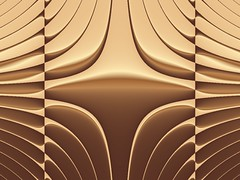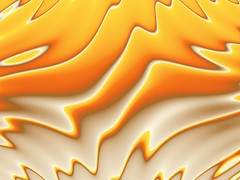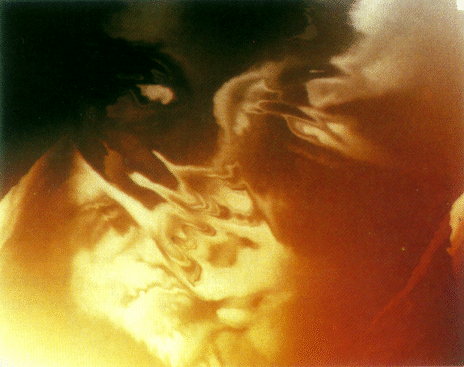# Genetic Art – Part 5

I have written a quick PDF paper describing the processes I have used for creating Genetic Art.

Here are some examples of Genetic Art animations on YouTube. If you have the bandwidth definitely check out the HD versions as they are far superior and show much more detail.

Jason.

# Genetic Art – Part 4

After some research into binary expression trees, infix, prefix and postfix I was able to create a new Genetic Art 3 mode for Visions Of Chaos.

Basing the image formulas on expression trees allows far more complex images to be bred using mutations, combinations and crossover.

Too see a full range of images this new Genetic Art method is capable of see this flickr set.

Here are a few thumbnails and the formulas that create them.((((y+x)*arctan((x*round(pi))))%((((y+x)*arctan((y/int(pi))))-cos((x+5.4)))%(((y%y)-y)%(2.7*4.5))))+((((y+x)*arctan((y/int(pi))))-cos((x+5.4)))%(((y%y)-y)%(2.7*4.5))))(((pi%4.2)*arctan(((x*y)+sin((y*tan(7.2))))))*((((pi%4.2)*arctan(((x*y)+sin((y*tan(7.2))))))%(((y-sin(y))%(x*sqrt(pi)))-(((1.2-pi)%sgn((x/4.2)))*abs((x+y)))))-((pi%4.2)*arctan(((x*y)+sin((y*tan(7.2))))))))(((((1.3/0.9)+sin((y*x)))%(((1.3/0.9)+sin((y*x)))%(((y*0.5)%6.5)-cos((x%(y-sqr(pi)))))))+((((1.3/0.9)+sin((y*x)))-(((1.3/0.9)+sin((y*x)))%(((y*0.5)%6.5)-cos((x%(y-sqr(pi)))))))*(((1.3/0.9)+sin((y*x)))%(((y*0.5)%6.5)-cos((x%(y-sqr(pi))))))))+((((1.3/0.9)+sin((y*x)))%(((1.3/0.9)+sin((y*x)))%(((y*0.5)%6.5)-cos((x%(y-sqr(pi)))))))+((((1.3/0.9)+sin((y*x)))-(((1.3/0.9)+sin((y*x)))%(((y*0.5)%6.5)-cos((x%(y-sqr(pi)))))))%(((y+round(pi))%(x+cos(y)))+int(((x/7.4)*(y-(7.4*sin(7.2)))))))))

There is also a high-def video of Genetic Art animations here. The animations change the digit nodes in the expression tree slightly over each frame.

Jason.

# Genetic Art – Part 3

picbreeder is an amazing example of genetic art online that allows anyone to evolve existing images or start from scratch and evolve their own. See here for some great examples.

picbreeder is the first genetic art system I have seen that has results beyond the usual abstract output of genetic art software and can actually produce images that “look like something” (even though in some cases it is clearly Pareidolia). Here are a few example images (there are thousands more in their gallery);The back end uses neural networks that mutate and breed that control the images. The results are very inspiring.

After a long waste of time most interesting experiment with the website these two are examples of what I evolved.If you want to be distracted (in a good way) for a while give it a try. Everyone that contributes increases the gene pool of images. So be your own God artist.

There is also a nice collection of genetic art links here.

Jason.

# Genetic Art – Part 2

After my initial Genetic Art success I was inspired to try a formula based approach.

For Genetic Art 2 the process of creation is as follows;
1. The screen pixels are mapped to X and Y values. The default X and Y coordinates range from -5 to +5.
2. Every coordinate/pixel is fed through the current formula and all the pixel values are scaled within the range of 0-255. This allows each pixel to be assigned a color from the currently loaded color palette.

Here are a few thumbnail images and their associated formulas;arctan((y)+y-sin(y)+arctan(pi)+tan(x*y)/sin(x))y-arctan((y)-arctan(y-x)-sin(y*pi)-sqr(y)*y-sin(y*x-0.3))arctan(((y)+tan((x+y)-sin(x+pi)-sin(x*y/pi)*sin(((y*x+pi))))))

Even though I am still only mutating formulas (and not breeding 2 parent formulas) the results using a formula based approach are much more varied.

If you would like to try making your own Genetic Art, try Visions Of Chaos.

There are many more examples of what the Genetic Art 2 process can create here.

Stay tuned for Genetic Art 3. I still want to have a true tree based approach to the formulas so they can be cross-bred and mutated. So two or more parents can be combined to create similar images that have attributes of both of them. A nice example of this methodology is shown here.

Jason.

# Genetic Art – Part 1

Genetic art is an interesting concept. Create a computer program that takes simple genes and combines them to create art.

One of the original people to kick off the genetic art (or as he calls it evolutionary art) revolution was Karl Sims. His art was created by combing relatively simple formulas and allowing human observers to select images they found pleasing. From this repeated process the formulas that had good ratings would evolve and mutate to become the parents of the future generations.

For example this imagewas created using the formula
(+ (min 10.8 (warp-rel image image (bump image x 9.6 #(0.57 0.02 0.15) #(0.52 0.03 0.38) 3.21 2.49 10.8))) (dissolve #(0.81 0.4 0.16) x (dissolve y #(0.88 0.99 0.66) image)))

Being inspired by that work I gave Genetic Art a go myself. The basic principle/algorithm is as follows;
1. Each image is created from a “rule string” or genetic code of a series of characters between A-Z and a-z. Each letter represents a formula.
2. Take a grid of pixels (an image) and assign each pixel a value of 1.
3. The image has X and Y coordinates between (for example) -3 and +3.
4. Each pixel coordinates is fed through a series of formulas (based on the rule string) to find a final pixel value.
5. This is repeated for every pixel in the image.
6. The values calculated for each pixel are then scaled between 1 and 255 so they can be mapped to a color palette.

For example the rule AXiQI generates the imageIn this initial version of Genetic Art I only used mutation of images, and not breeding. So for example if the above image was mutated one of the rule string characters would be randomly changed to simulate genetic mutation.

More examples of what this form of Genetic Art can produce can be seen here.

If you are interested in trying a hands on Genetic Art mutation yourself download Visions Of Chaos and have a go.

Jason.